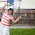## Sunday, August 28, 2016

### Bonetrousle - HackerRank world code sprint #6 - Time complexity

August 28, 2016

Worked on the algorithm over 3 hours, Bonetrousle. HackerRank code sprint #6.

Julia started to train herself to get smart on algorithm problem solving - contest level. First and big lesson is to put the time complexity analysis first - she experienced the pain and valued the lesson she learned through 3+ hours.

Editorial notes from HackerRank:

https://www.hackerrank.com/contests/world-codesprint-6/challenges/bonetrousle/editorial

Will work on this blog later!

Come back on Sept. 1, 11:15pm.

The problem is about loops, and let us work on the example used in the editorial notes:

N = 15, K = 8, B =3, in other words, find 3 distinct numbers from set {1, 2, ..., 8}, and the sum is 15.

First idea: brute force one
Brute force, 3 number, each one is chosen from 1-8, 3 time, then, simple combinatorics: 8 * 7 * 6, K (K-1)(K-2), , the order does not matter, so it is should C(8,3).

As we know, N < 10^18, K<=10^18, so, Julia, you like to make any points, try to avoid any brute force solution like the above.

Second idea: How low it can be?
Find B numbers, as we know, from the example,
N = 15, K = 8, B = 3, there are more than 1 solution.

But, there is one definitely taking minimum time. That is to find maximum number in B number set first, and it takes O(1) time. Then, in decreasing order, find one by one. The total time can be  small as O(B).

Simple math, we can analyze first if N is in the range of minimum value and maximum value range first.

if B > K, not possible;

Assuming B <= K,
Minimum value = 1 + 2 +... +B,
Maximum value = K + (K-1) + (K-B+1)

#### 2 comments:

1.I got it differently. I start from "minimum boxes selection". In given example it would be [1, 2, 3]. I know it has totally 6 pastas. I need 15 pastas. I get the difference - 9 pastas i this case. I divide difference by the number of boxes (rounding down), and add result to each box. So i have [4, 5, 6] which is acceptable result. Sometimes there is remainder from division - say we need 17 pastas. Our minimum box selection give 6, difference is 11. This divided by 3 gives 3 and remainder 2.
Then I share remainder (1 for each) between top boxes. In this case it gives [4, 6, 7] which is proper solution.

Right now I cant see where it could fail...but it fails (runtime error) cases from 7 upwards on HR :( Even though it gives proper results when ran locally on given data.

1.Give the following the counter example:
Given N = 20, K = 8, B =3, in other words, find 3 distinct numbers from set {1, 2, ..., 8}, and the sum is 20.

Given 20,

20 -6 = 14, since 1+2 +3 = 6,
then,

5 5 4

1 2 3

6 7 7 = 20

-----

How can you get from {6, 7, 7} to one of solutions: {5, 7, 8}